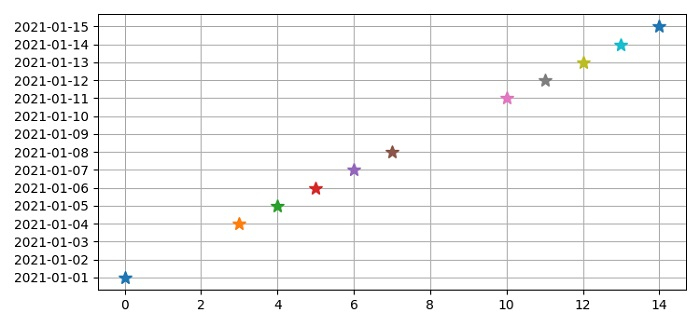# How to skip empty dates (weekends) in a financial Matplotlib Python graph?

To skip weekends in a financial graph in matplotlib, we can iterate the time in dataframe and skip the plot if weekday is 5 or 6.

## Steps

• Set the figure size and adjust the padding between and around the subplots.

• Create a dataframe with keys time.

• Iterate zipped index and time of a date frame.

• If iterated timestamp is having weekday 5 or 6, don't plot them.

• Other than 5 or 6 weekday, plot the points.

• Set the current tick locations of Y-axis.

• Lay out a plot with grid lines.

• To display the figure, use show() method.

## Example

import pandas as pd
from matplotlib import pyplot as plt
plt.rcParams["figure.figsize"] = [7.50, 3.50]
plt.rcParams["figure.autolayout"] = True
df = pd.DataFrame(dict(time=list(pd.date_range(start="2021-01-01", end="2021-01-15"))))
for i, t in zip(df.index, df.time):
if t.weekday() in (5, 6):
pass
else:
plt.plot(i, t, marker="*", ms=10)
plt.yticks(df.time)
plt.grid(True)
plt.show()

## Output